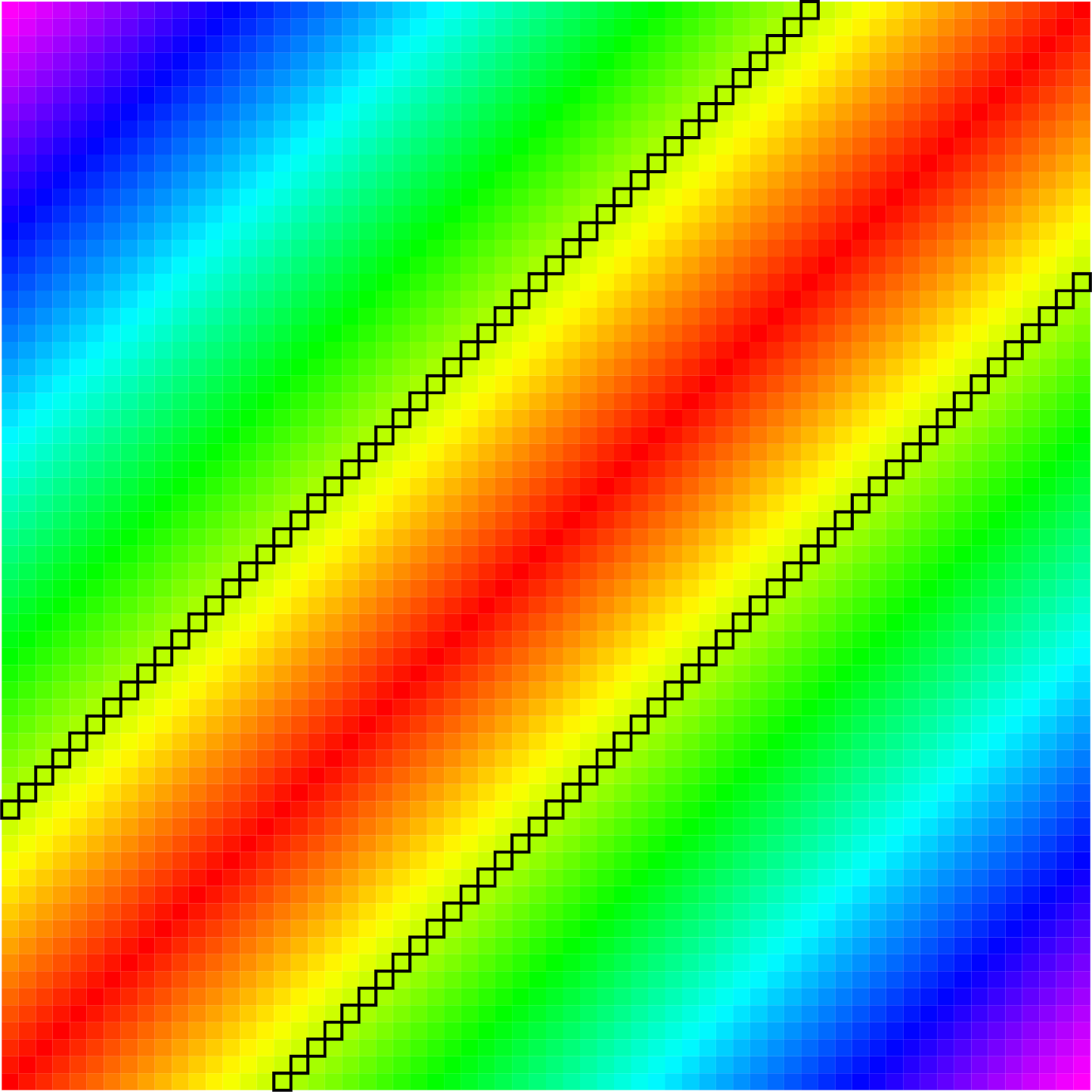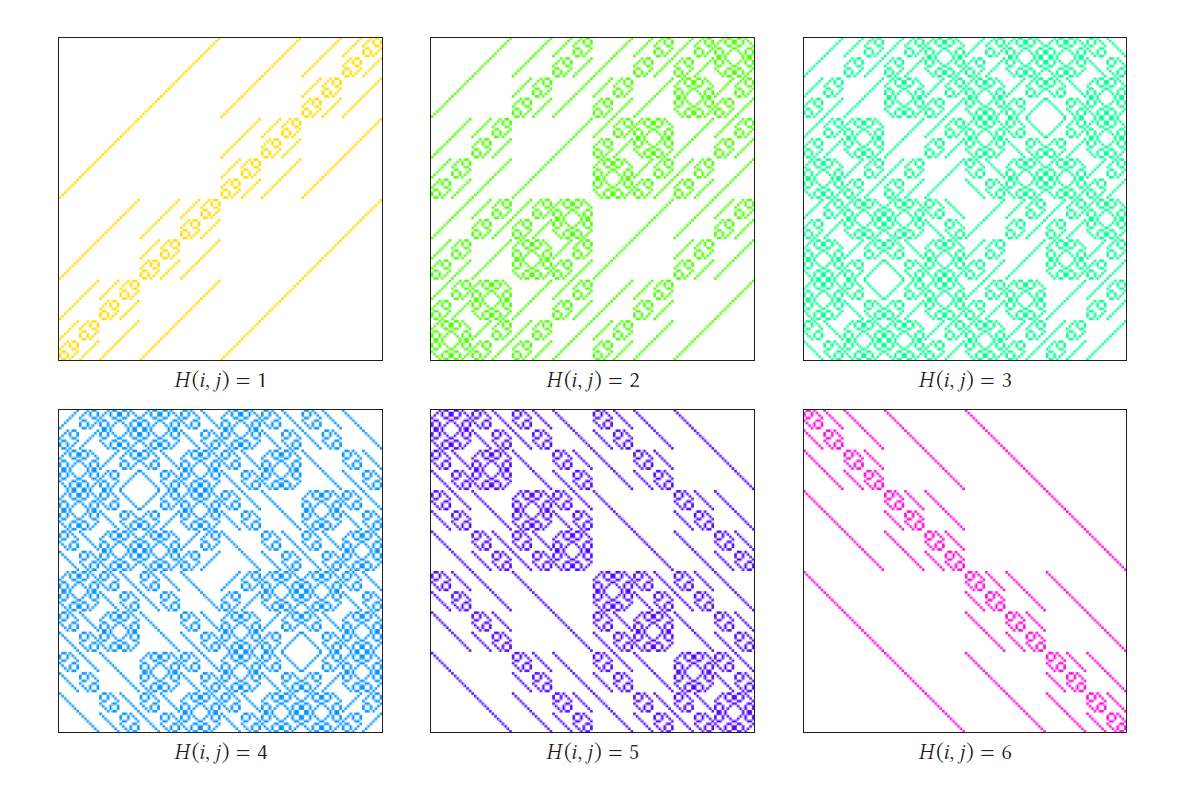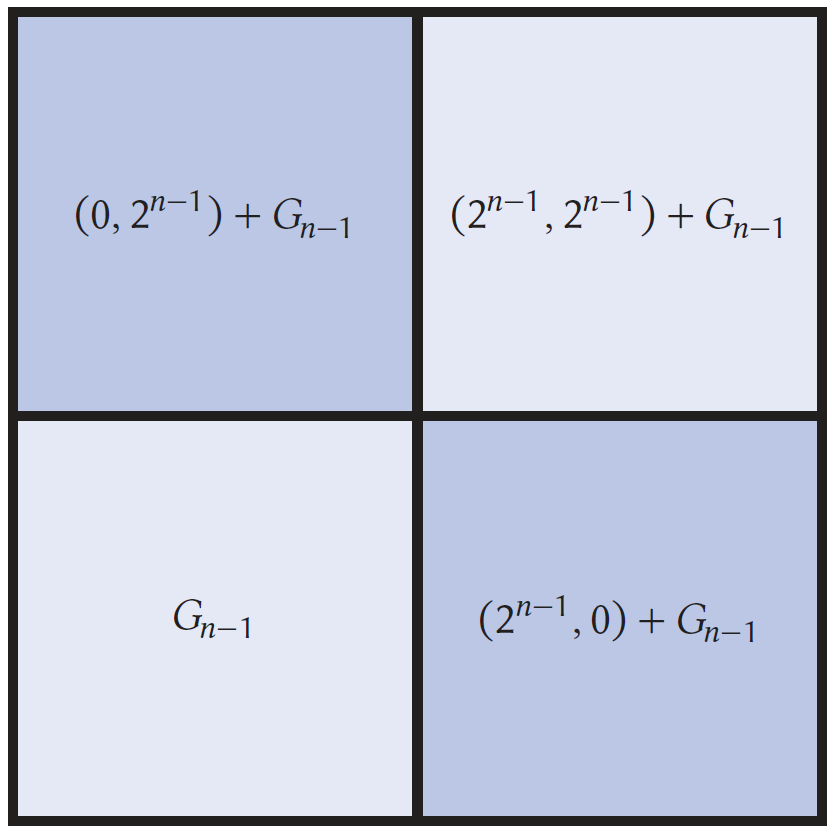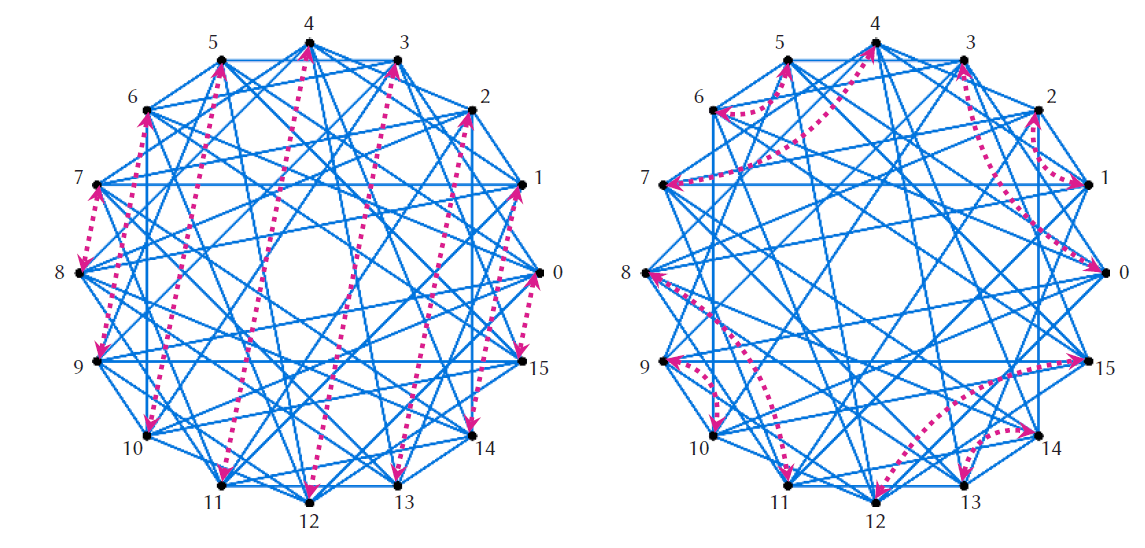# The hidden harmonies of Hamming distance

Chris Boucher explores the secrets and symmetries behind a measure of the distance between binary stringsWe think about the concept of distance all the time—how far is it to go visit a friend, is my phone close enough to the router to get wifi?—but in mathematics there are many ways of measuring the distance between things.

Mathematics is sometimes thought to be a world of dense, dry formulae and esoteric logic, but its structures, especially when paired with modern computing, can generate images of arresting complexity like the one above. The goal of this article is to introduce and explore these images, which arise from attempts to visualise a notion of distance on the integers called Hamming distance.

A distance function assigns a non-negative number to any pair of objects to serve as a measure of how far apart they are. In order to qualify as a distance, such an assignment needs three key features. First, the distance from an object to itself is zero. Second, the distance from object $x$ to object $y$ is always the same as the distance from object $y$ to object $x$. Finally, a path from one object to another that goes through a third is never shorter than the direct path between the two objects.

More formally, if we denote the distance from $x$ to $y$ by $D(x,y)$, then for any object $z$, $$D(x,y)\leq D(x,z)+D(z,y).$$ In what follows, we will consider two different ways of measuring the distance between positive integers.

## Euclidean distance and Hamming distance

The most common way to measure the distance between two numbers is using Euclidean distance, with which you may already be familiar. Given two numbers $x$ and $y$, the Euclidean distance between them, expressed as $E(x,y)$, is simply $\vert x-y\vert$.

In the mid-twentieth century, the American mathematician Richard Hamming developed the Hamming distance to measure the distance between sequences of zeros and ones by counting the number of positions in which the sequences differ, a measure that has the three features necessary for a distance. So, for example, the Hamming distance between 10110 and 11110 is 1 as they only differ in the second position from the left—these strings are close.

Working in the early days of computer science, Hamming used this distance measure in his analysis of the effectiveness of error detection and correction in the transmission of messages encoded by strings of zeros and ones. The number of errors an encoding could correct was related to how ‘spread out’ the space of code words was as measured by Hamming distance. Hamming distance can also be thought of as a distance on the integers by using binary representations.

For example, \begin{align*} 22 & = 1 {\color{gray}\times 2}^4 + 0 {\color{gray}\times 2}^3 + 1 {\color{gray}\times 2}^2 + 1 {\color{gray}\times 2}^1 + 0 {\color{gray}\times 2}^0 = 10110_2,\\ 30 & = 1 {\color{gray}\times 2}^4 + 1 {\color{gray}\times 2}^3 + 1 {\color{gray}\times 2}^2 + 1 {\color{gray}\times 2}^1 + 0 {\color{gray}\times 2}^0 = 11110_2. \end{align*} Here, the subscript $2$ indicates that the number is in base 2 rather than base 10. Writing $H(x,y)$ for the Hamming distance between two positive integers $x$ and $y$, $H(22,30)=1$ because the binary representations of 30 and 22 differ in one position. Of course, the Euclidean distance is $E(22,30) = \vert 22-30\vert=8$.

The binary representation of the number six, $110_2$, has only three digits, but we can measure the distance from 6 to a number like 22 whose binary representation has five digits by padding with extra zeros on the left, which has no effect on the underlying number. So $6 = 110_2=00110_2$, and $H(6,22) = H(00110_2, 10110_2) = 1.$ Hamming distance clearly measures distance between positive integers quite differently than the standard Euclidean distance does. For example, $H(22,25)=4$, so 22 is much further from 25 than it is from 6 as measured by Hamming distance, but 22 is much closer to 25 than to 6 on the number line. While we can ‘see’ the Euclidean distance between two numbers in their separation on the number line, it is not immediately clear how or whether we can visualise Hamming distance.

## Euclidean distance in a squareEuclidean distance visualised in a square. If $E(i,j)=E(i’,j’)$ then $(i,j)$ and $(i’,j’)$ are the same colour; cells with $E(i,j) =16$ are outlined.

Distance is a function of two variables, so let us try to visualise $E(i,j)$ and $H(i,j)$ as the pair $(i,j)$ ranges over a grid of integer pairs. We’ll define $B_n$ to be the set of positive integers whose binary representation contains $n$ or fewer digits, so ${B_n = \left\{ 0,1,\dots, 2^n-1\right\}}$.

Our first strategy to visualise Euclidean and Hamming distance is to use the grid $G_n = \{(i,j)\mid i,j\in B_n \}$ as our canvas and give each different value of $E(i,j)$ a different colour. For Euclidean distance, this gives a fairly simple picture. Points $(i,j)$ that satisfy $E(i,j)=k$ lie either on the line $y=x+k$ or $y=x-k$, and so the points whose coordinates are at Euclidean distance $k$ consist of two parallel lines. On the right, the squares are $128\times 128$ cells, with the cell at position $(i,j)$ corresponding to that point in the grid.

While this picture is fairly simple, there are some features worth noting. First, the image is symmetric about the line $y=x$ because $E(x,y) = E(y,x)$, a symmetry that would be present for any distance measure. Second, the image is unchanged if we translate along the line $y=x$ because for any $z$, \begin{equation} \label{EuclideanSymmetry}\tag{?} E(x+z, y+z) = E(x,y). \end{equation}

## Hamming distance in a square

Using the same strategy to visualise Hamming distance produces more interesting images. The picture below shows a few colourings of the $128 \times 128$ grid $G_7$ using different values of the Hamming distance between cell coordinates. Colouring all the points in the grid $G_9$ according to the Hamming distance between their coordinates gives the image at the start of the article.

One thing to notice is that there are only $n$ possible values for the Hamming distance between two points in $B_n$, so there are only $n$ colours used in the grid, whereas there are $2^n$ possible values for the Euclidean distance between two such points. What is interesting about this picture is the nested structure of the grids $G_n$—the image is a fractal in that the same patterns recur at progressively smaller scales.The fractal looks like a quilt made of squares of similar structure. Moving from a point $(i,j)$ in some sub-square of the grid to a similarly positioned point in another sub-square involves translating one or both components by a power of two. To explore this, let’s use the notation $x+A$ for the set of numbers  in the set $A$ shifted by $x$. So formally, $x+A = \{x+a\mid a\in A \}$, and, for example, $3+\{8,9,10\} = \{11,12,13\}$. This notation allows us to recognise $B_{n}$ as consisting of $B_{n-1}$ together with $2^{n-1} + B_{n-1}$. For example: $B_2 = \left\{ 0,1,2,3 \right\} \quad \textrm{and} \quad B_3 = \left\{ 0,1,2,3,0+2^2, 1+2^2, 2+2^2, 3+2^2 \right\}.$ This means that the grid $G_{n}$ is built from four quadrants that are shifts of $G_{n-1}$ (depicted below right).If $i\in B_{n-1}$, then the binary representation of $i+2^{n-1}$ is identical to that of $i$ except for having a 1 in the $2^{n-1}$th place instead of a 0. It follows that if $j$ is also in $B_{n-1}$, then $i+2^{n-1}$ and $j$ differ in all the same places $i$ and $j$ do, with an additional difference in the $2^{n-1}$th place. If, for example, $i=01101_2$, $j=00111_2$ where $i,j\in B_5$, then $i+2^5=101101_2$. Stated more precisely: \begin{equation} \label{HammingShiftEquation}\tag{⭐} H(i+2^{n-1}, j) = H(i,j) + 1. \end{equation} This means that the cells in the lower right quadrant will have a colour value of exactly one more than the cell in the corresponding position in the lower left quadrant. A similar calculation shows that \begin{equation} \label{HammingShiftEquation2}\tag{?} H(i, j+2^{n-1}) = H(i,j) + 1. \end{equation} In a similar way, the upper left quadrant has the same relationship to the lower left quadrant as the lower right quadrant does. In particular, the upper left and lower right quadrants have identical colouring. If $i,j\in B_{n-1}$, then $i+2^{n-1}$ and $j+2^{n-1}$ both have a 1 in the $2^{n-1}$th place and differ in precisely the same places as $i$ and $j$. If we again take $i=01101_2$, $j=00111_2\in B_5$, then $i+2^5=101101_2$ and $j+2^5=100111_2$. That is, \begin{equation} \label{HammingShiftEquation3}\tag{?} H(i+2^{n-1}, j+2^{n-1}) = H(i,j). \end{equation} This means the colouring of the upper right block will be identical to that of the lower left block. Since this block structure holds for $n=1,2,\dots$, we can build the Hamming fractal starting with $G_1$. The first few steps are shown below:The nested structure illustrated here causes the fractal nature of the grids $G_n$ to become increasingly apparent as $n\to \infty$. For larger values of $n$, the images contain finer detail and more complex self-similarity. In fact,  Equations (⭐) to  (?) which cause the structure in these images, have generalisations that involve a type of addition called the XOR sum.

## The block structure and XOR sums

Hamming distance counts the number of positions in which the binary representations of $i$ and $j$ differ and is closely related to the binary operation of XOR addition. The XOR sum of two natural numbers $i$ and $j$, which we will denote $i\oplus j$, is the natural number whose binary representation has a 1 in any position where the binary representations of $i$ and $j$ differ and a 0 in any position where they are the same. For example, $\begin{array}{l l | l l} & 61 & & 111101_2 \\ \oplus & 15 & \oplus & 001111_2 \\ \hline & 50 & & 110010_2 \end{array}$ If we use the notation $\Vert i \Vert$ for the number of ones in the binary representation of $i$, then $\Vert i \Vert = H(0,i)$. The binary representation of $i\oplus j$ has as many 1s as positions where the binary representations of $i$ and $j$ differ, so $H(i,j) = \Vert i\oplus j \Vert$. Because the binary representation of $2^{n-1}$ contains all zeros except for a one in the $2^{n-1}$ position, for any $i$, $i\oplus 2^{n-1}$ will have an identical binary representation to $i$ except in the $2^{n-1}$ position, where it will differ.

Another way of thinking of the block structure in the images in the previous section is in terms of the $\oplus$ operation. If $i,j\in B_{n-1}$, so both $i$ and $j$ have zeros to the left of the $2^{n-2}$th place in their binary representations, then adding $2^{n-1}$ to either number affects their binary representations only by adding a one in the $2^{n-1}$th place. That is, equations (⭐) and (?) can be generalised if $i+2^{n-1}$ is replaced by $i\oplus 2^{n-1}$, so that it becomes $\Vert (i\oplus 2^{n-1}) \oplus j \Vert = \Vert i\oplus j \Vert+1\quad \textrm{and} \quad \Vert i \oplus (j\oplus2^{n-1}) \Vert =\Vert i\oplus j \Vert+1.$ Moreover equation (?) generalises to become $\Vert (i\oplus2^{n-1})\oplus (j\oplus2^{n-1}) \Vert =\Vert i+j \Vert$.

Equations (⭐) to (?), as well as giving rise to this nested structure, can also be generalised using the XOR sum to explain more intricate symmetries of this image. Suppose the numbers $i$ and $j$ agree at some position of their binary expansions like $i =01\mathbf{1}01_2$ and $j=10\mathbf{1}10_2$, which agree in the third position. Then ‘$\oplus$-ing’ the same number to both doesn’t change this agreement. If, for example, $a=10100_2$, then $i\oplus a = 11\mathbf{0}01_2$ and $j\oplus a = 00\mathbf{0}11_2$ both also agree in this position.

If, on the other hand, $i$ and $j$ disagree at the some position of their binary expansions like $i =01\mathbf{0}01_2$ and $j=10\mathbf{1}10_2$, which disagree in the third position, then ‘$\oplus$-ing’ the same number to both doesn’t change this disagreement either. Here, $i\oplus a = 11\mathbf{1}01_2$ and $j\oplus a = 00\mathbf{0}11_2$ both also disagree in this position. Thus, the binary expansions of $i\oplus a$ and $j \oplus a$ differ in precisely the same positions as the binary expansions of $i$ and $j$, so \begin{equation} \label{HammingSymmetry}\tag{?} H(i\oplus a, j\oplus a) = H(i,j). \end{equation} Comparing this to equation (?), we see that the operation $\oplus$ is to Hamming distance much as the operation of ordinary addition is to Euclidean distance.

## Visualisations on a circle

We can get an alternative visualisation by identifying the points of $B_n$ with equally spaced points around a circle, and connecting points that are a given distance apart.

We place the number 0 at the three o’clock position on the circle and spread the numbers $0,1,\dots, 2^n-1$ evenly around the circle anticlockwise. If we connect points that correspond to numbers that are Hamming distance $k$ from one another, we get some interesting images. The image on the right corresponds to $n=4$ and $k=2$ and the image below to $n=6$ and $k=3$.

The relationship in equation (?) illuminates many symmetries of these images. If we ‘$\oplus$-shift’ all the points on the circle, that is, if we move every vertex $i$ to the vertex $i\oplus a$ for some $a$, then we don’t change any of the connections because such a shift doesn’t change Hamming distance.

For example, if $a=2^n-1$, the binary representation of $a$ consists of $n$ ones, and the binary representation $x\oplus a$ has ones precisely where that of $x$ has zeros, and has zeros precisely where that of $x$ has ones. That is, $x\oplus a = a-x$, and the image is symmetric with respect to the interchange of $x$ and $a-x$, which amounts to a reflection over a line through the centre of the circle.

In the image (below left) purple arrows connect each point $x$ with the point $a-x$. Choosing a different value of $a$, say $a=3$, then interchanging $x$ and $x\oplus a$ corresponds to a more subtle symmetry of the graph as shown on the right.Symmetry with respect to swapping $x$ with $x\oplus (2^n-1)=15-x$ (left), and $x$ with $x\oplus 3$ (right).

## Where can we go from here?We have unlocked some, but by no means all of the mysteries presented by images like the Hamming fractal, and circle visualisations. There are many symmetries left to uncover, and fascinating questions to ask about these images. Why is there a white circle at the centre? How is the size of this circle related to $n$ and the chosen Hamming distance? Why does there seem to be more white space toward the centre of the circle pictures? What can be said about the structure of the white space around the centre circle, for example the eight ovals surrounding it?While it is not at all obvious from the circle picture for $n=4$, $k=2$, this graph actually has two connected components shown on the right. Is it always the case for these graphs? If not, under what conditions is it the case? Can the number of connected components be described in terms of the numerical properties of the vertex numbers they contain? When do these graphs have other interesting properties; for example, when are they bipartite? Just two examples to illustrate the variety of circle virtualisations are below.

The circle visualisations for $B_7$ if $H(i,j)=5$ (left), and $B_8$ if $H(i,j)=2$ (right).

The Wolfram demonstration projects by Enrique Zeleny (2014) and Chris Boucher (2020) are freely available and offer a platform for quickly generating some of these images. I hope you share my enthusiasm for these pictures and will feel inspired to explore them yourself.Chris teaches and learns at Salem State University in Salem, Massachusetts, USA. He has interest and some expertise in probability, mathematical computing, and data visualisation. He also has interest, but less expertise, in basketball and home improvement.